## Central Angles and Arcs

There are several different angles associated with circles. Perhaps the one that most immediately comes to mind is the central angle. It is the central angle's ability to sweep through an arc of 360 degrees that determines the number of degrees usually thought of as being contained by a circle.

Central angles are angles formed by any two radii in a circle. The vertex is the center of the circle. In Figure 1, ∠ AOB is a central angle.Figure 1 A central angle of a circle.

## Arcs

An arc of a circle is a continuous portion of the circle. It consists of two endpoints and all the points on the circle between these endpoints. The symbol is used to denote an arc. This symbol is written over the endpoints that form the arc. There are three types of arcs:

• Semicircle: an arc whose endpoints are the endpoints of a diameter. It is named using three points. The first and third points are the endpoints of the diameter, and the middle point is any point of the arc between the endpoints.
• Minor arc: an arc that is less than a semicircle. A minor arc is named by using only the two endpoints of the arc.
• Major arc: an arc that is more than a semicircle. It is named by three points. The first and third are the endpoints, and the middle point is any point on the arc between the endpoints.

In Figure 2AC is a diameter.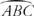is a semicircle.Figure 2 A diameter of a circle and a semicircle.

In Figure 3is a minor arc of circle P.Figure 3 A minor arc of a circle.

In Figure 4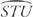is a major arc of circle Q.Figure 4 A major arc of a circle.

Arcs are measured in three different ways. They are measured in degrees and in unit length as follows:

• Degree measure of a semicircle: This is 180°. Its unit length is half of the circumference of the circle.
• Degree measure of a minor arc: Defined as the same as the measure of its corresponding central angle. Its unit length is a portion of the circumference. Its length is always less than half of the circumference.
• Degree measure of a major arc: This is 360° minus the degree measure of the minor arc that has the same endpoints as the major arc. Its unit length is a portion of the circumference and is always more than half of the circumference.

In these examples, mindicates the degree measure of arc ABlindicates the length of arc AB, andindicates the arc itself.

Example 1: In Figure 5, circle O, with diameter AB has OB = 6 inches. Find (a) mand (b) l.Figure 5 Degree measure and arc length of a semicircle.is a semicircle. m= 180°.

Sinceis a semicircle, its length is half of the circumference.Postulate 18 (Arc Addition Postulate): If B is a point on, then m+ m= m.

Example 2: Use Figure 6 to find m( m= 60°, m= 150°).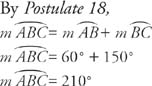Figure 6 Using the Arc Addition Postulate.

Example 3: Use Figure of circle P with diameter QS to answer the following.

a. Find mb. Find mc. Find m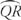d. Find m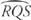Figure 7 Finding degree measures of arcs.

a. m(The degree measure of a minor arc equals the measure of its corresponding central angle.)

b.= 180° (is a semicircle.)

c. m= 130°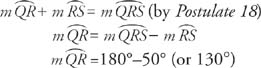d. m= 310° (is a major arc.) The degree measure of a major arc is 360° minus the degree measure of the minor arc that has the same endpoints as the major arc.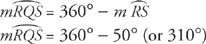The following theorems about arcs and central angles are easily proven.

Theorem 68: In a circle, if two central angles have equal measures, then their corresponding minor arcs have equal measures.

Theorem 69: In a circle, if two minor arcs have equal measures, then their corresponding central angles have equal measures.

Example 4: Figure 8 shows circle O with diameters AC and BD. If m ∠1 = 40°, find each of the following.Figure 8 A circle with two diameters and a (nondiameter) chord.a. m= 40° (The measure of a minor arc equals the measure of its corresponding central angle.)

b. m= 40° (Since vertical angles have equal measures, m ∠1 = m ∠2. Then the measure of a minor arc equals the measure of its corresponding central angle.)

c. m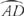= 140° (By Postulate 18m+ m= mis a semicircle, so m+ 40° = 180°, or m= 140°.)

d. m ∠ DOA = 140° (The measure of a central angle equals the measure of its corresponding minor arc.)

e. m ∠3 = 20° (Since radii of a circle are equal, OD = OA. Since, if two sides of a triangle are equal, then the angles opposite these sides are equal, m ∠3 = m ∠4. Since the sum of the angles of any triangle equals 180°, m∠3 + m ∠4 + m ∠ DOA = 180°. By replacing m ∠4 with m ∠3 and m ∠ DOA with 140°,f. m ∠4 = 20° (As discussed above, m ∠3 = m ∠4.)